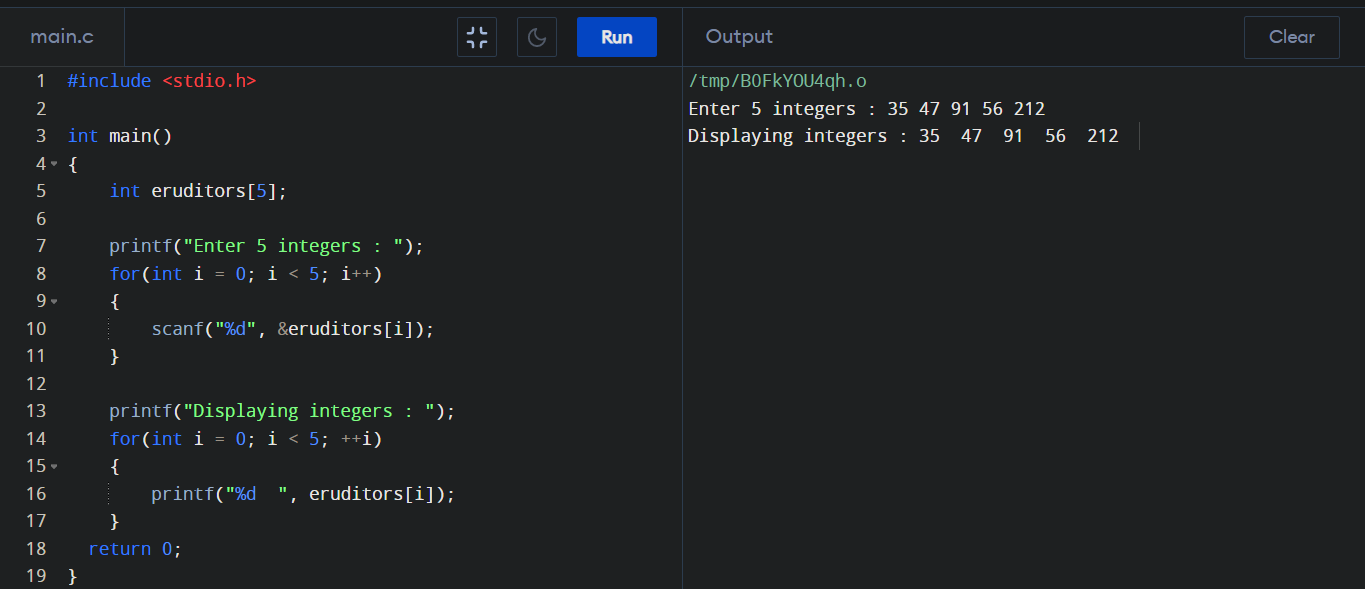### What is Array ?

An array is a collection of similar data items stored at contiguous memory locations and the array elements can be accessed randomly using index of that array. In simple word an array is a variable that can store multiple values of same data type.

### Why do we need array ?

We use variables to store data. But sometime when we store more than one data by declaring variables every time (like - x1, x2, x3...) it becomes complicated. And for this reason, we use Array, where we can store a large amount of data by declaring the variable only once.

### How to declare an array ?

###### Syntax :
``````
data_type array_name[array_size];
``````
###### Example :
``````
// Here, we are declaring an array, eruditors, of integer type. And its size is 25.
// Array declaration by specifying size.

int eruditors;

// Array declaration by user specified size.

int n = 25;
int eruditors[n];
``````

### How to initialize an array?

``````
//It is possible to initialize an array during declaration. For example,

int eruditors = {35, 98, 48, 117, 56};

//We can also initialize an array without specifying the array size. The compiler knows its size is 3 as we are initializing it with 3 elements. For example,

int eruditors[] = {211, 101, 169};
``````

### Array Input/Output Program in C :

``````
//Program to take 5 values from the user and store them in an array.
//Print the elements stored in the array.

#include <stdio.h>

int main()
{
int eruditors;

printf("Enter 5 integers : ");
for(int i = 0; i < 5; i++)
{
scanf("%d", &eruditors[i]);
}

printf("Displaying integers : ");
for(int i = 0; i < 5; ++i)
{
printf("%d  ", eruditors[i]);
}
return 0;
}
``````
##### Output :### Accessing Array Elements:

A specific element in an array is accessed by an integer index. Array index starts with 0 and goes till size of Array minus 1.
``````
int eruditors[] = {211, 101, 169};

//Here 211, 101 and 169 can be accessed by eruditors, eruditors and eruditors respectively.
``````

### How to Change Value of Array elements?

###### Syntax :
``````
array_name[index] = new_value;
``````
###### Example :
``````
int eruditors = {117, 104, 101, 98, 91}

//Make the value of the first element to 91 & the fifth element to 117

eruditors = 91;
eruditors = 117;
``````

### Key Points about Array :

• ~ Arrays can be used to store collection of primitive data types such as int, float, double, char etc.
• ~ The size and type of an array cannot be changed once it is declared.
• ~ Arrays have 0 as the first index, not 1.
• ~ If the size of an array is n, to access the last element, the n-1 index is used.
• ~ All arrays consist of contiguous memory locations. The lowest address corresponds to the first element and the highest address to the last element.

### Advantages of an Array in C :

• ~ Random access of elements using array index.
• ~ Use of less line of code as it creates a single array of multiple elements.